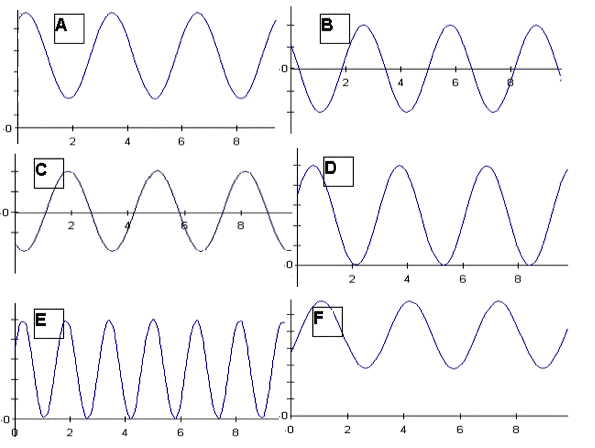Teaching Physics with the Physics Suite Edward F. Redish

Oscillating graphs

A mass is hanging from a spring off the edge of a table. The position of the mass is measured by a sonic ranger sitting on the floor 25 cm below the mass's equilibrium position. At some time, the mass is started oscillating. At a later time, the sonic ranger begins to take data.

Below are shown a series of graphs associated with the motion of the mass and a series of physical quantities. The graph labeled (A) is a graph of the mass's position as measured by the ranger. For each physical quantity identify which graph could represent that quantity for this situation. If none are possible, answer N.

 velocity of the mass net force on the mass force exerted by the spring on the mass kinetic energy of the mass potential energy of the spring gravitational potential energy of the massNot finding what you wanted? Check the Site Map for more information.